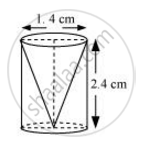Share

# From a solid cylinder whose height is 2.4 cm and diameter 1.4 cm, a conical cavity of the same height and same diameter is hollowed out - Mathematics

Course

#### Question

From a solid cylinder whose height is 2.4 cm and diameter 1.4 cm, a conical cavity of the same height and same diameter is hollowed out. Find the total surface area of the remaining solid to the nearest cm[Use π = 22/7]

#### SolutionGiven that,

Height (h) of the conical part = Height (h) of the cylindrical part = 2.4 cm

Diameter of the cylindrical part = 1.4 cm

Therefore, radius (r) of the cylindrical part = 0.7 cm

Slant height (l) of conical part = sqrt(r^2+h^2)

= sqrt((0.7)^2+(2.4)^2) =sqrt(0.49+5.76)

=sqrt(6.25) = 2.5

Total surface area of the remaining solid will be

= CSA of cylindrical part + CSA of conical part + Area of cylindrical base

= 2πrh + πrl + πr2

= 2xx 22/7xx 0.7xx2.4+22/7xx0.7xx2.5+22/7xx0.7xx0.7

= 4.4x2.4+2.2x2.5+2.2x0.7

=10.56+5.50+1.54 = 17.60 cm2

The total surface area of the remaining solid to the nearest cm2 is 18 cm2.

Is there an error in this question or solution?